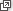## Motivation

Il y a quelque chose à compléter dans cette démonstration. Je n'ai pas le temps … Après cela, il y aura, j'espère, des gens qui trouveront leur profit à déchiffrer tout ce gâchis.

(There is something to complete in this proof. I do not have the time … Later, there will be, I hope, some people who will find it to their advantage to decipher all this mess.)

— Évariste Galois on polynomials, the night before his death, 29 May 1832.

By now we are familiar with linear and quadratic functions, i.e.,

$f(x) = ax + b \qquad \text{or} \qquad f(x) = ax^2 + bx + c,$

where $$a,b,c$$ are real constants. We know how to graph linear functions and how to solve linear equations $$ax+b=0$$. We also know how to draw graphs of quadratic functions (which are parabolas) and how to solve quadratic equations $$ax^2 + bx + c = 0$$.

It's then natural to consider a slightly more complicated function, with just one more term, an $$x^3$$ term,

$f(x) = ax^3 + bx^2 + cx + d.$

Here $$a,b,c,d$$ are real constants. The situation now becomes a bit more complicated. A cubic function's graph is not always of the same shape, and a cubic equation $ax^3 + bx^2 + cx + d = 0$ is more difficult to solve than a quadratic one. In secondary school mathematics such equations can only be solved in special cases. (We will give a sketch of a general method in the Links forward section.)

But why stop at cubic functions? Why not add an $$x^4$$ term? Why not an $$x^{3\,095\,283}$$ term? In general, if we allow any terms of the type $$a x^n$$, where $$a$$ is a real constant and $$n$$ a non-negative integer, then the functions we obtain are called polynomial functions. A general expression for a polynomial function is

$f(x) = a_n x^n + a_{n-1} x^{n-1} + a_{n-2} x^{n-2} + \dots + a_2 x^2 + a_1 x + a_0.$

The highest power $$n$$ occurring is called the degree, and $$a_0, a_1, \dots, a_n$$ are real numbers called the coefficients.

Some examples of polynomials are the functions

$f(x) = 3 x^{3} - \frac{11}{3} x + 42, \qquad g(x) = \sqrt{7} x^{3982} + 5 x^{13} - 8 \qquad \text{and} \qquad h(x) = 6.$

Polynomials are a natural type of function to consider: a generalisation of linear, quadratic and cubic functions. They can sometimes be solved exactly. Their graphs can be sketched. Many quantities in the real world are related by polynomial functions.

Importantly, any smooth function can be approximated by polynomials. So proficiency in dealing with polynomials is also important.

The reader should be aware of the module Polynomialsfor Years 9–10, which provides useful revision of some concepts in polynomials, and covers some interesting related topics. This module is designed to complement the Years 9–10 module.

Next page - Content - Some jargon